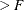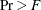The CANCORR Procedure

Displayed Output

If the SIMPLE option is specified, PROC CANCORR produces means and standard deviations for each input variable. If the CORR option is specified, PROC CANCORR produces correlations among the input variables. Unless the NOPRINT option is specified, PROC CANCORR displays a table of canonical correlations containing the following:

• Canonical Correlations. These are always nonnegative.

• Adjusted Canonical Correlations (Lawley, 1959), which are asymptotically less biased than the raw correlations and can be negative. The adjusted canonical correlations might not be computable, and they are displayed as missing values if two canonical correlations are nearly equal or if some are close to zero. A missing value is also displayed if an adjusted canonical correlation is larger than a previous adjusted canonical correlation.

• Approx Standard Errors, which are the approximate standard errors of the canonical correlations

• Squared Canonical Correlations

• Eigenvalues of INV(E)*H, which are equal to CanRsq/(1–CanRsq), where CanRsq is the corresponding squared canonical correlation. Also displayed for each eigenvalue is the Difference from the next eigenvalue, the Proportion of the sum of the eigenvalues, and the Cumulative proportion.

• Likelihood Ratio for the hypothesis that the current canonical correlation and all smaller ones are zero in the population. The likelihood ratio for all canonical correlations equals Wilks’ lambda.

• Approx F statistic based on Rao’s approximation to the distribution of the likelihood ratio (Rao 1973, p. 556; Kshirsagar 1972, p. 326)

• Num DF and Den DF (numerator and denominator degrees of freedom) and Pr(probability level) associated with the F statistic

Unless you specify the NOPRINT option, PROC CANCORR produces a table of multivariate statistics for the null hypothesis that all canonical correlations are zero in the population. These statistics, as described in the section Multivariate Tests in Chapter 4: Introduction to Regression Procedures, are as follows:

• Wilks’ lambda

• Pillai’s trace

• Hotelling-Lawley trace

• Roy’s greatest root

For each of the preceding statistics, PROC CANCORR displays the following, depending on the specification of the MSTAT= option.

If you specify MSTAT=FAPPROX (also the default value), the following statistics are displayed:

• an F approximation or upper bound

• Num DF, the numerator degrees of freedom

• Den DF, the denominator degrees of freedom

•, the probability level

If you specify MSTAT=EXACT, the following statistic is displayed:

• a t value

Unless you specify the SHORT or NOPRINT option, PROC CANCORR displays the following:

• both Raw (unstandardized) and Standardized Canonical Coefficients normalized to give canonical variables with unit variance. Standardized coefficients can be used to compute canonical variable scores from the standardized (zero mean and unit variance) input variables. Raw coefficients can be used to compute canonical variable scores from the input variables without standardizing them.

• all four Canonical Structure matrices, giving Correlations Between the canonical variables and the original variables

If you specify the REDUNDANCY option, PROC CANCORR displays the following:

• the Canonical Redundancy Analysis (Stewart and Love, 1968; Cooley and Lohnes, 1971), including Raw (unstandardized) and Standardized Variance and Cumulative Proportion of the Variance of each set of variables Explained by Their Own Canonical Variables and Explained by The Opposite Canonical Variables

• the Squared Multiple Correlations of each variable with the first m canonical variables of the opposite set, where m varies from 1 to the number of canonical correlations

If you specify the VDEP option, PROC CANCORR performs multiple regression analyses with the VAR variables as dependent variables and the WITH variables as regressors. If you specify the WDEP option, PROC CANCORR performs multiple regression analyses with the WITH variables as dependent variables and the VAR variables as regressors. If you specify the VDEP or WDEP option and also specify the ALL option, PROC CANCORR displays the following items. You can also specify individual options to request a subset of the output generated by the ALL option; or you can suppress the output by specifying the NOPRINT option.

SMC

Squared Multiple Correlations and F Tests. For each regression model, identified by its dependent variable name, PROC CANCORR displays the R square, Adjusted R square (Wherry, 1931), F Statistic, and. Also for each regression model, PROC CANCORR displays an Approximate 95% Confidence Interval for the population R square Helland (1987). These confidence limits are valid only when the regressors are random and when the regressors and dependent variables are approximately distributed according to a multivariate normal distribution. The average R squares for the models considered, unweighted and weighted by variance, are also given.

CORRB

Correlations Among the Regression Coefficient Estimates

STB

Standardized Regression Coefficients

B

Raw Regression Coefficients

SEB

Standard Errors of the Regression Coefficients

CLB

95% confidence limits for the regression coefficients

T

T Statistics for the Regression Coefficients

PROBT

Probability > |T| for the Regression Coefficients

SPCORR

Semipartial Correlations between regressors and dependent variables, Removing from Each Regressor the Effects of All Other Regressors

SQSPCORR

Squared Semipartial Correlations between regressors and dependent variables, Removing from Each Regressor the Effects of All Other Regressors

PCORR

Partial Correlations between regressors and dependent variables, Removing the Effects of All Other Regressors from Both Regressor and Criterion

SQPCORR

Squared Partial Correlations between regressors and dependent variables, Removing the Effects of All Other Regressors from Both Regressor and Criterion